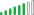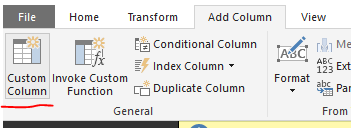cancel
Showing results for
Did you mean:New Member

## Calculated column based on date fields

I have a requirement to create a calculated measure either in desktop in report or in the query. How can we achieve this using if then, also at report only measure can be used to create a measure it doesnot allow me to use date columns:

(CASE WHEN (FACT_ORDER.ORDER_ACTUAL_END_DATE > FACT_ORDER.ORDER_SCHED_END_DATE) THEN (FACT_ORDER.ORDER_ACTUAL_END_DATE - FACT_ORDER.ORDER_SCHED_END_DATE) ELSE 0 END)

Any help is appreciated

2 ACCEPTED SOLUTIONSSolution Supplier

Hey @kmuir.

Are you trying to return a number value or a date?

The below code can be used in a "Calculated Column" to achieve this as a number:

`IF(FACT_ORDER.ORDER_ACTUAL_END_DATE > FACT_ORDER.ORDER_SCHED_END_DATE, (FACT_ORDER.ORDER_ACTUAL_END_DATE - FACT_ORDER.ORDER_SCHED_END_DATE), 0)`

Make sure the data type of the new column is a number.

Hope this helps,

AlanMicrosoft

@kmuir

What @alanhodgson and @JoeSQL suggetsed is DAX, you can also do it with Power Query. Just add a custom column in Query Editor.`= if [FACT_ORDER.ORDER_ACTUAL_END_DATE] > [FACT_ORDER.ORDER_SCHED_END_DATE] then [FACT_ORDER.ORDER_ACTUAL_END_DATE] - [FACT_ORDER.ORDER_SCHED_END_DATE] else 0`

For more details, see: Power Query if Statements.

Regards,

5 REPLIES 5Solution Supplier

Hey @kmuir.

Are you trying to return a number value or a date?

The below code can be used in a "Calculated Column" to achieve this as a number:

`IF(FACT_ORDER.ORDER_ACTUAL_END_DATE > FACT_ORDER.ORDER_SCHED_END_DATE, (FACT_ORDER.ORDER_ACTUAL_END_DATE - FACT_ORDER.ORDER_SCHED_END_DATE), 0)`

Make sure the data type of the new column is a number.

Hope this helps,

AlanFrequent Visitor

ColumnName = IF(FACT_ORDER.ORDER_ACTUAL_END_DATE > FACT_ORDER.ORDER_SCHED_END_DATE, FACT_ORDER.ORDER_ACTUAL_END_DATE - FACT_ORDER.ORDER_SCHED_END_DATE,0)New Member

Should I add this code to query editor using Advance Editor. In report I am unable to add this logic as a new measure? Also in dax this syntax may not work. I need to knowcorrect syntax for adding to query editor as a new column.Microsoft

@kmuir

What @alanhodgson and @JoeSQL suggetsed is DAX, you can also do it with Power Query. Just add a custom column in Query Editor.`= if [FACT_ORDER.ORDER_ACTUAL_END_DATE] > [FACT_ORDER.ORDER_SCHED_END_DATE] then [FACT_ORDER.ORDER_ACTUAL_END_DATE] - [FACT_ORDER.ORDER_SCHED_END_DATE] else 0`

For more details, see: Power Query if Statements.

Regards,Solution Supplier

The formulas above are DAX, but I think you need to do this in MDX and use the IIF function (see here for details).

I am not a MDX expert so I can't write it for you, but I found some helpful links.

A similiar question was asked and answered here with the MDX provided.

Please post the end result if you figure it out so other can use it.

Thanks,

Alan